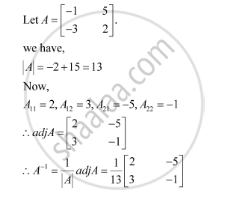Share

# Find the Inverse of Each of the Matrices (If It Exists). [(-1,5),(-3,2)] - CBSE (Commerce) Class 12 - Mathematics

#### Question

Find the inverse of each of the matrices (if it exists).

[(-1,5),(-3,2)]

#### SolutionIs there an error in this question or solution?

#### Video TutorialsVIEW ALL 

Solution Find the Inverse of Each of the Matrices (If It Exists). [(-1,5),(-3,2)] Concept: Adjoint and Inverse of a Matrix.
S